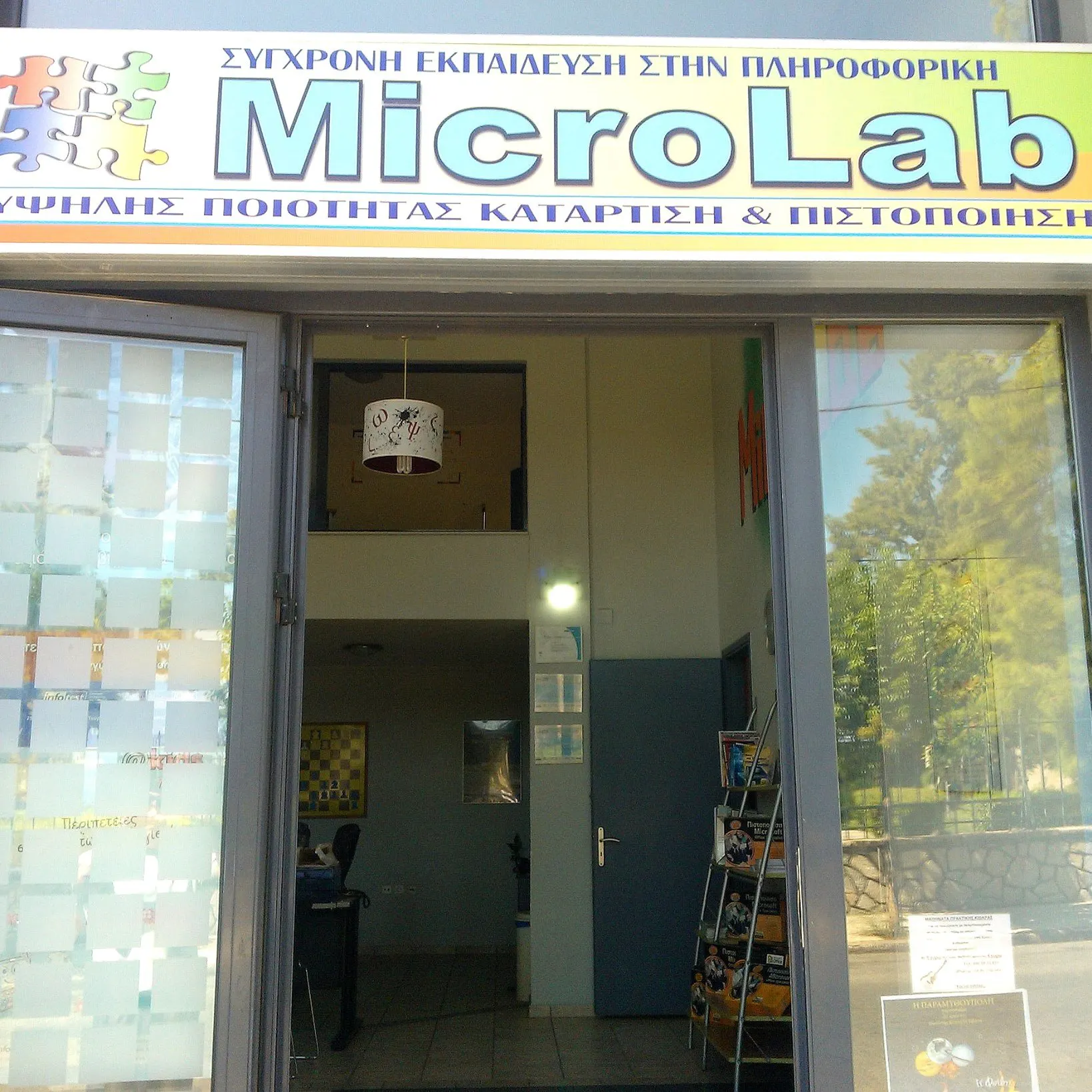Published

# Arduino Two Ultrasonic Sensor Radar - 360 degrees Rotation

We present you the radar we created in the MicroLab lab. This radar uses 2 ultrasonic sensors and 1 motor moving continuously 360 degrees.

ExpertShowcase (no instructions)20,267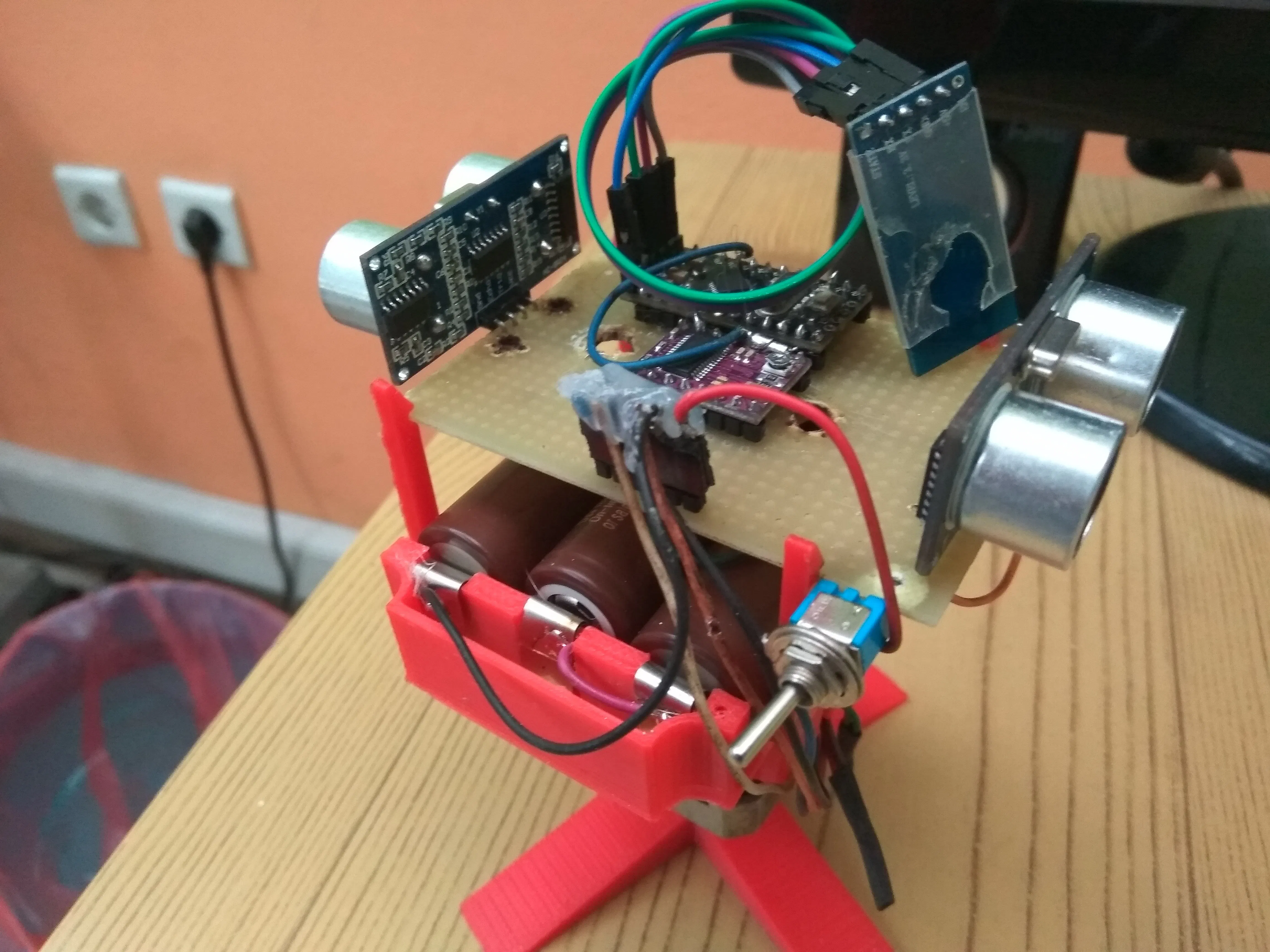## Things used in this project

### Hardware components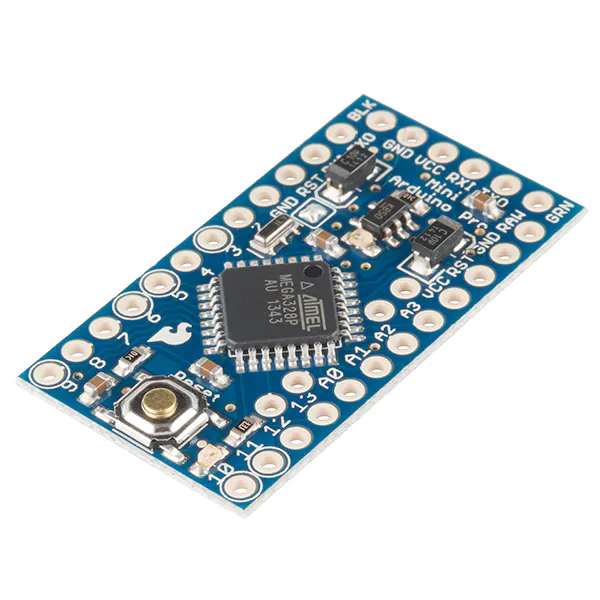SparkFun Arduino Pro Mini 328 - 5V/16MHz
×1Ultrasonic Sensor - HC-SR04 (Generic)
×2
 Sony 3V Lion battery 3000mAh
×3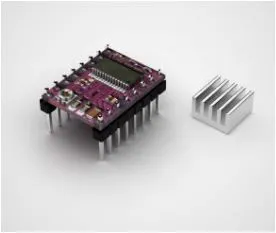Driver DRV8825 for Stepper Motors for Theremino System
×1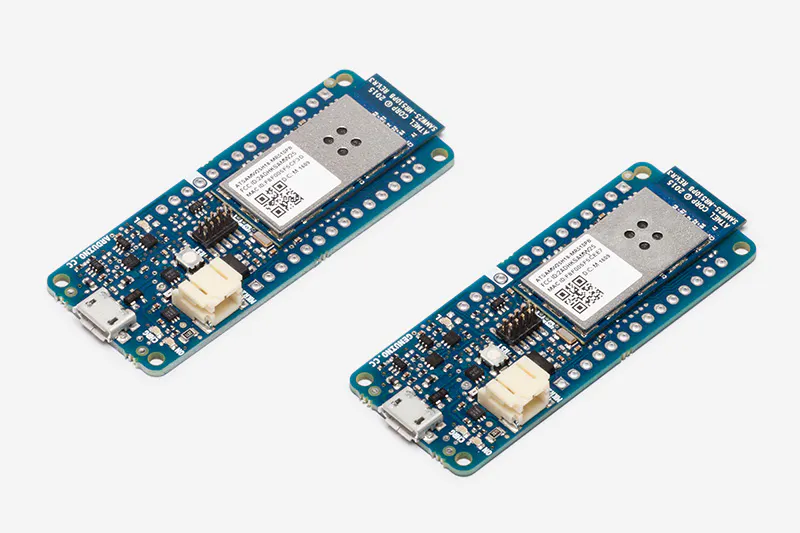Arduino MKR1000
×1
 DC motor (generic)
×1

### Software apps and online services

 Processing 3Arduino IDE

## Custom parts and enclosures

### 2-phased stepper motor of the Microcon

The motor has regulated for continiously rotation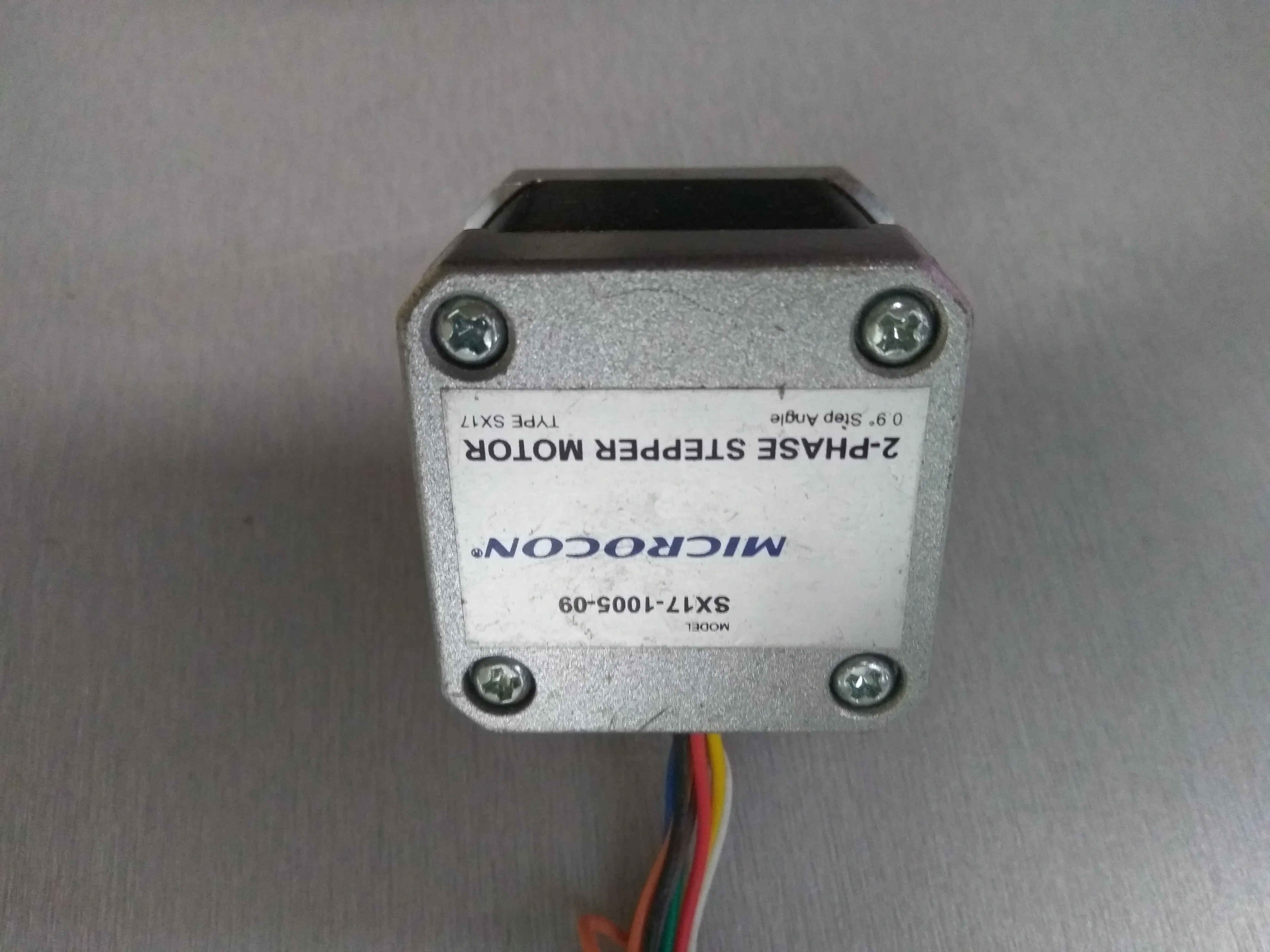### Arduino mini pro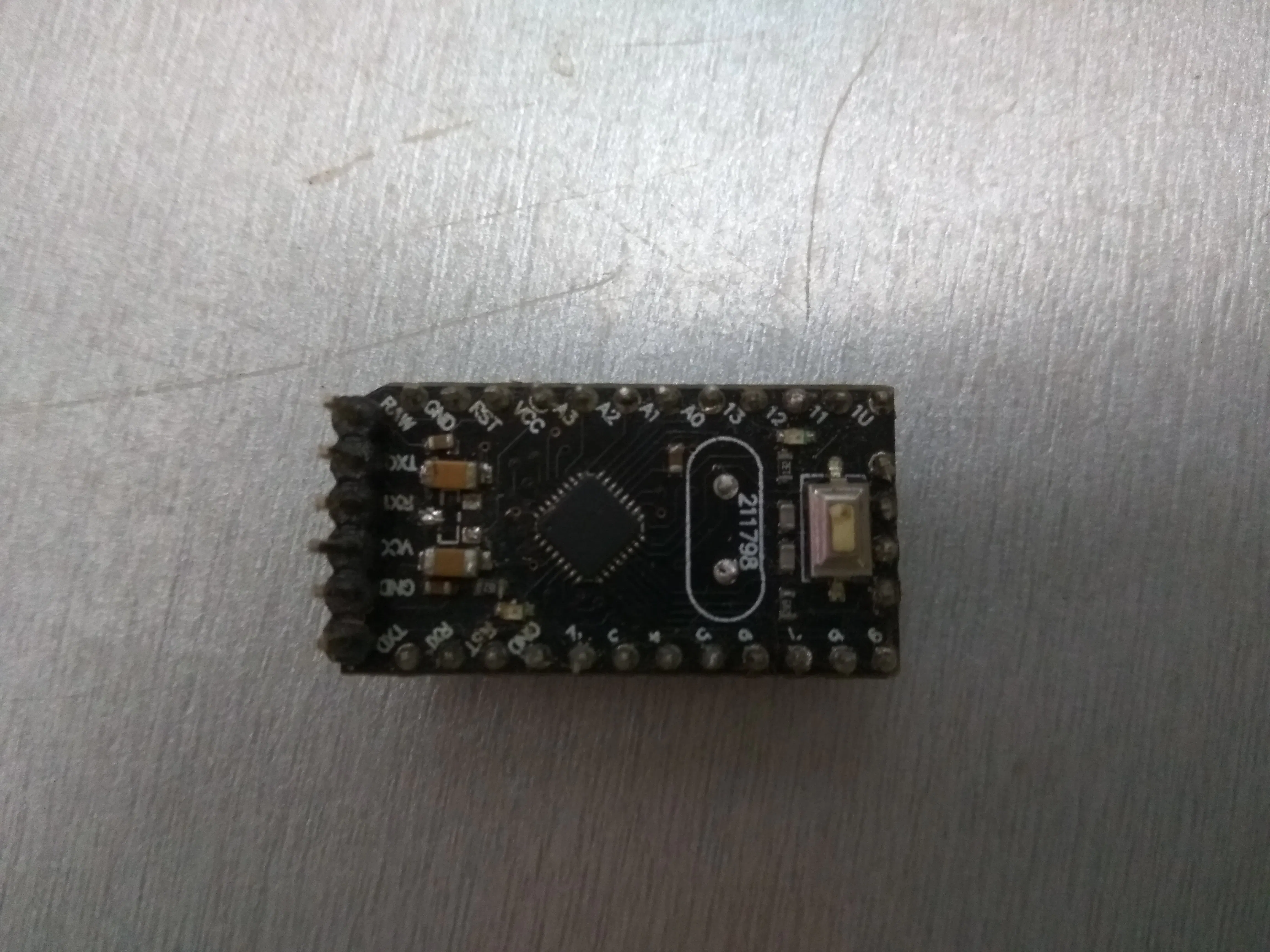### DRV8825 Stepper Motor Driver Carrier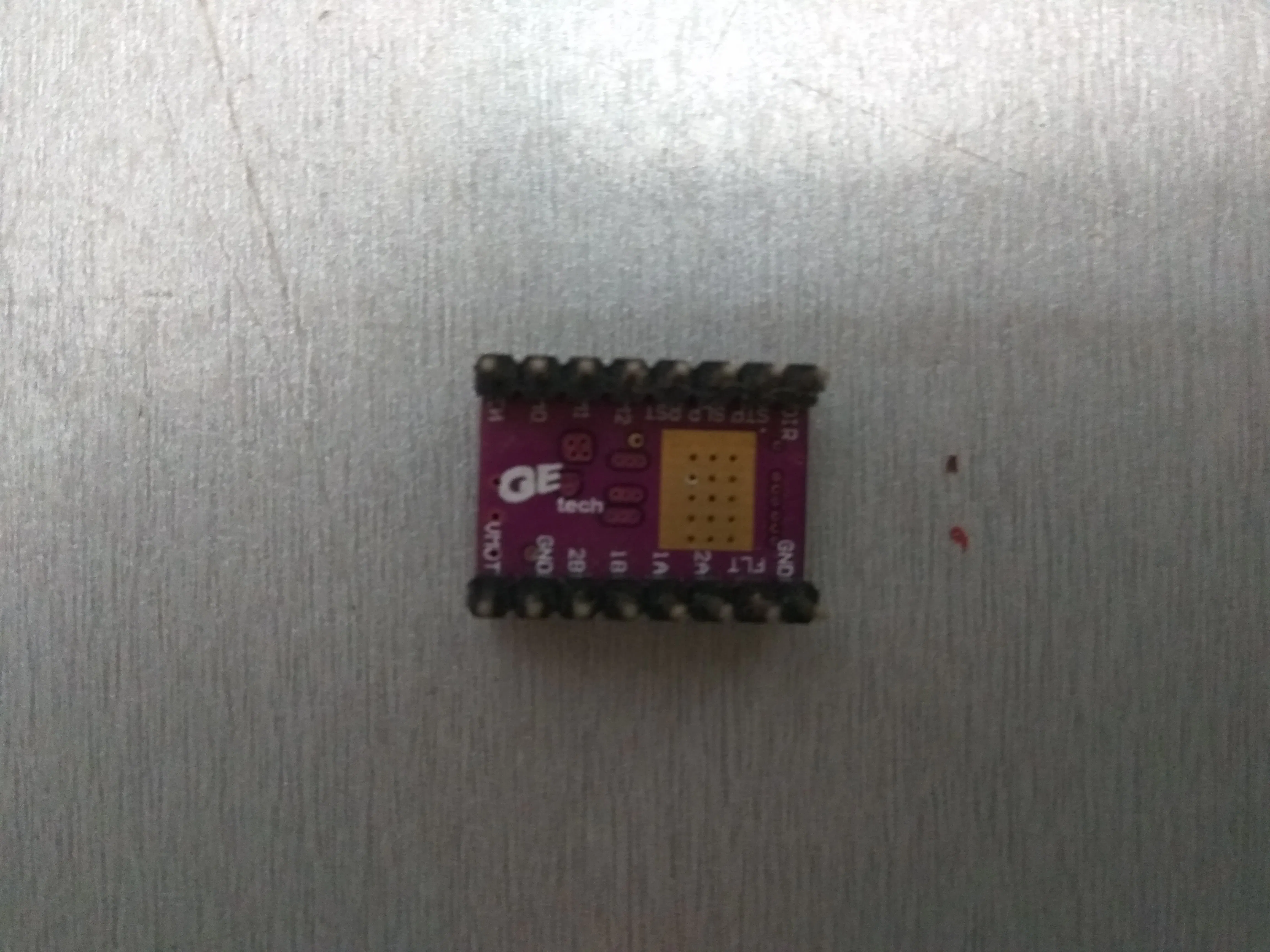### Plastics

All the plastic parts have made via 3D printer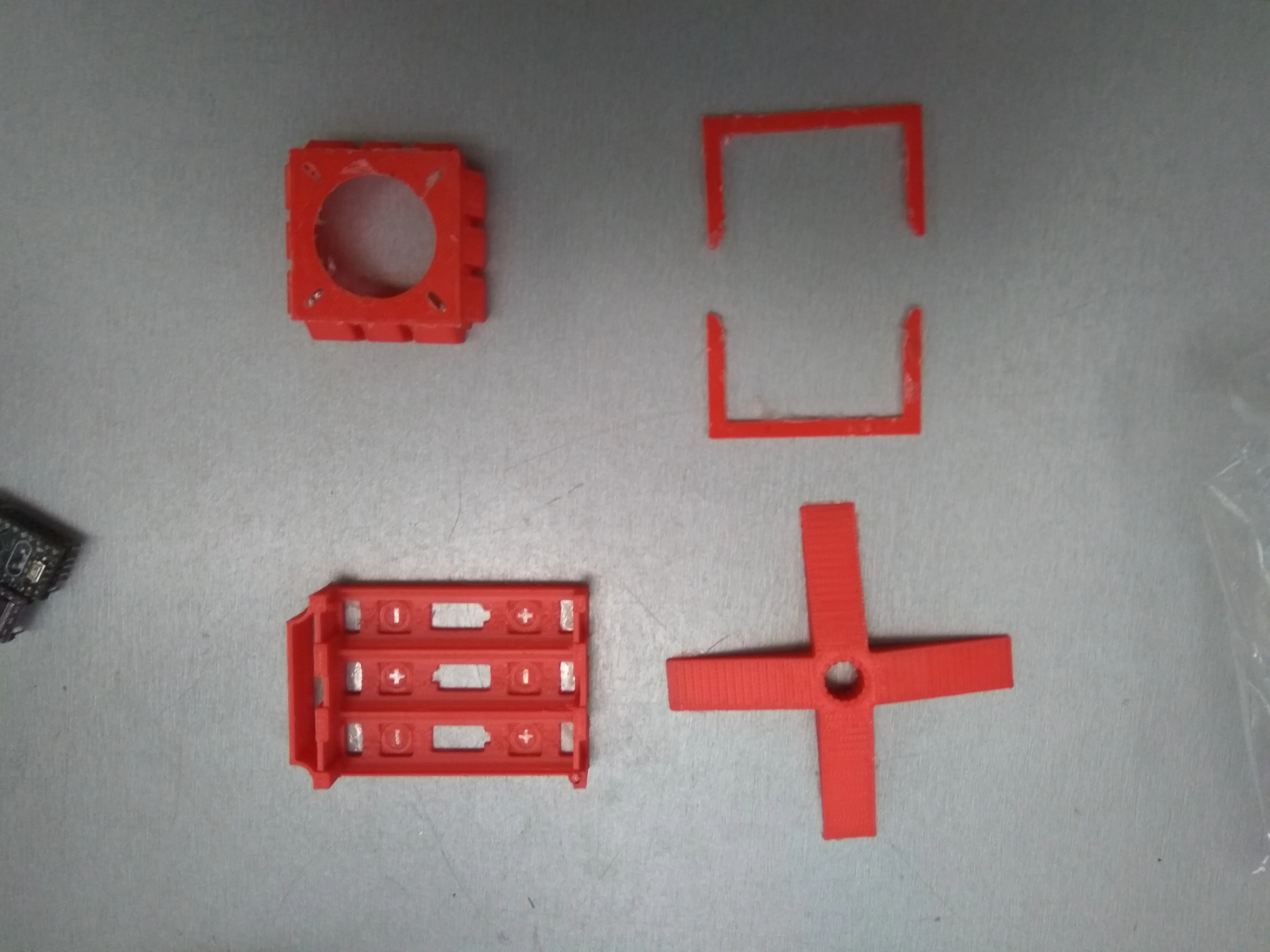### Batteries Lion 3000mAh each

The batteries are rechargeable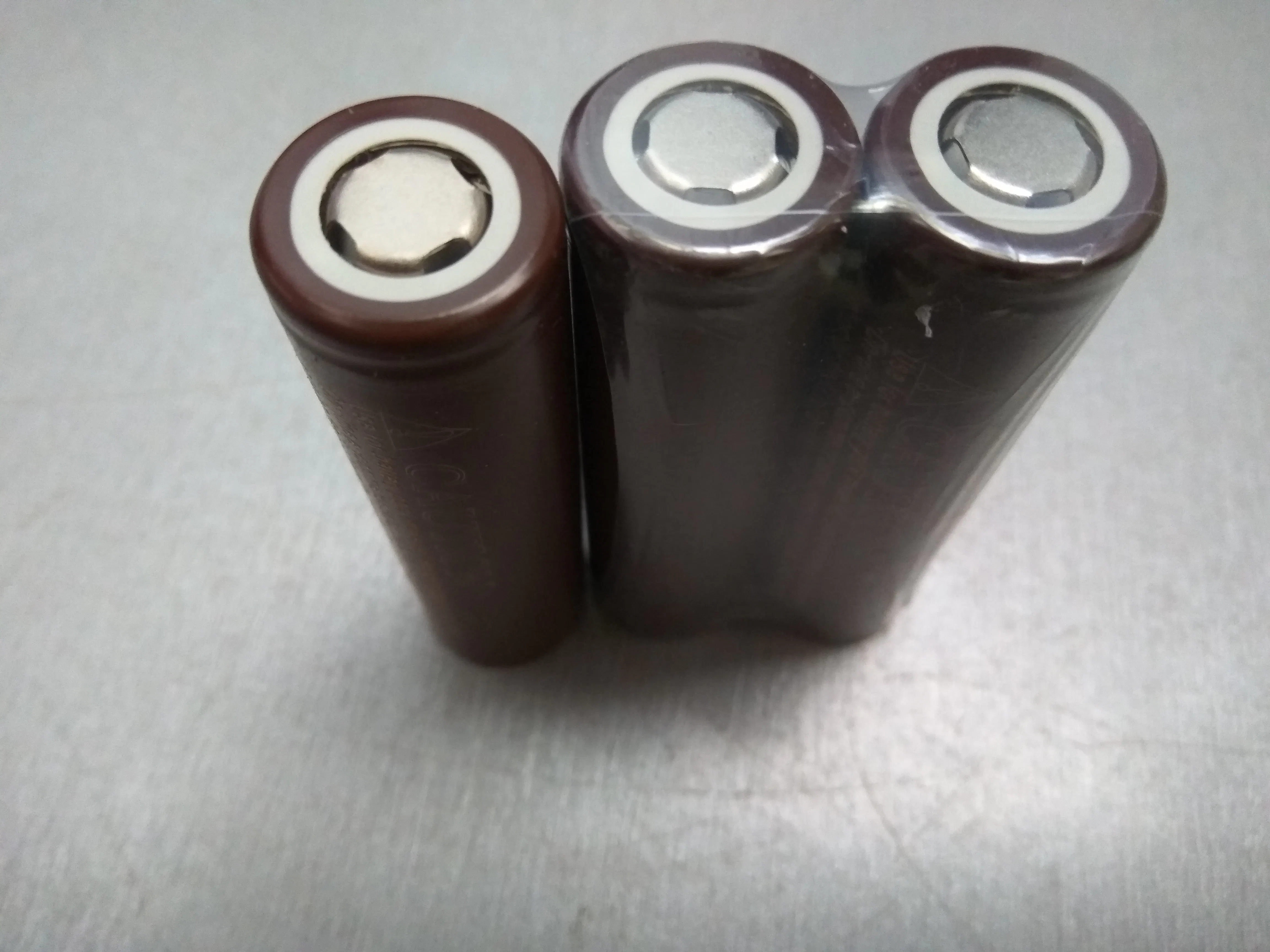### ultrasonic distance sensors for Arduino

Radar uses two (2) ultrasonic sensors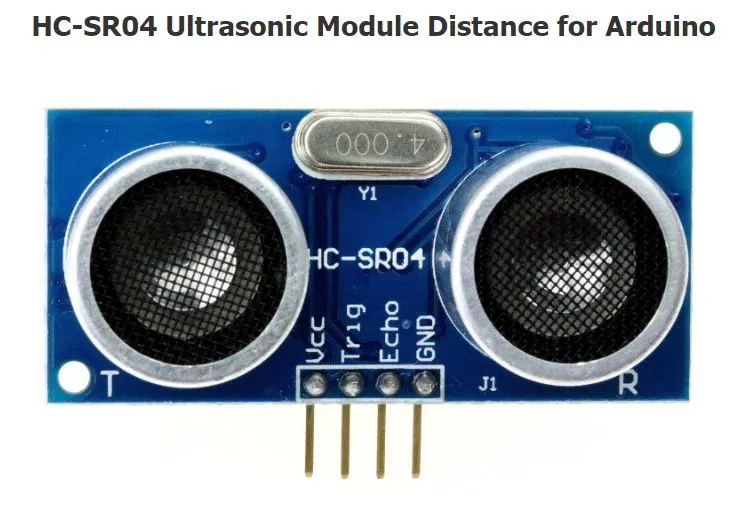### HC-05 6 Pin Wireless Bluetooth RF Transceiver Module Serial For Arduino

Radar gets the data via bluetooth communication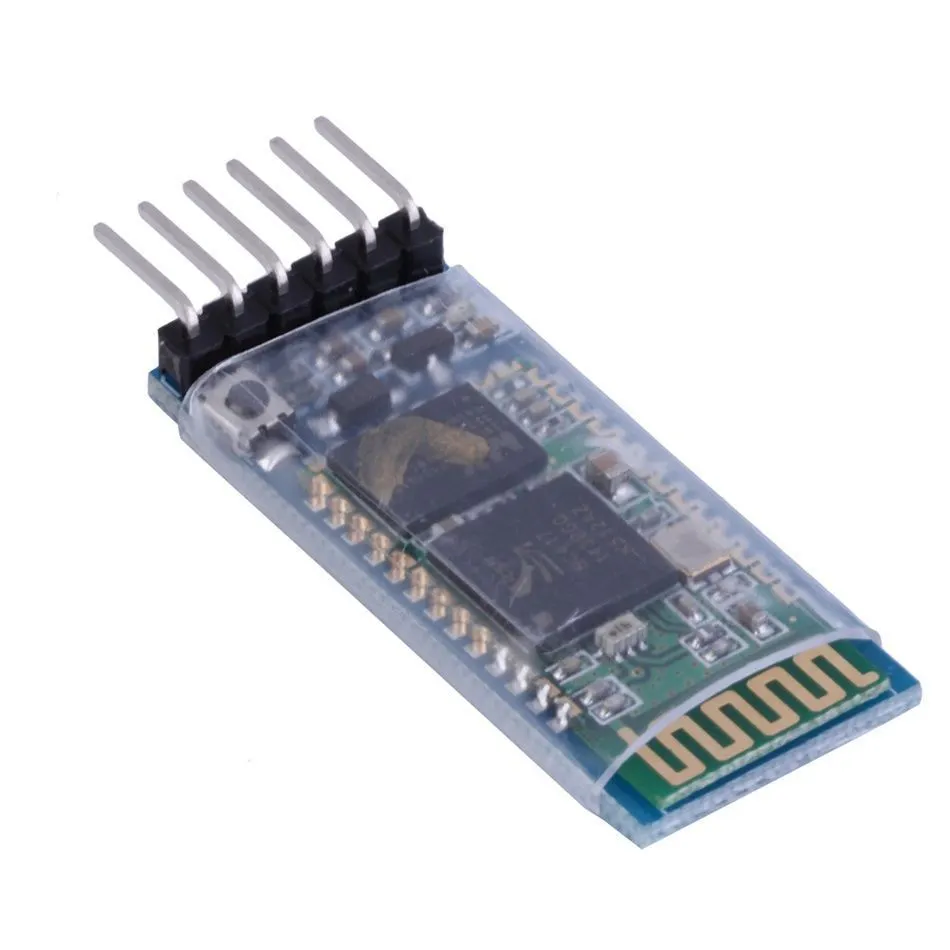## Code

### The whole MicroLab Radar code

Processing
```//this is the whole code running via Processing 3.3.6. (MicroLab-Greece)
//All parts tests carried out in Arduino IDE. The plastic parts are made via 3D printer
import processing.serial.*; //import library in order serial interface data
import java.awt.event.KeyEvent; //import library for reading data from serial port
import java.io.IOException;
Serial myPort;//defines serial object
//variable definition
String angle="";
String distance="";
String data="";
String noObject;
float pixsDistance;
int iAngle, iDistance;
int index1=0;
int index2=0;
PFont orcFont;

void setup() {

size (860, 860);

smooth();
myPort = new Serial(this,"COM5", 57600); //starting communication with specofic port
myPort.bufferUntil('.'); //reads data from port delimited by '.'

}

void draw() {

fill(98,245,31);
//simulation of moving line (motion and fade)
noStroke();
fill(0,4);
rect(0, 0, width, height);
fill(7,246,23);
drawLine();
drawObject();
drawText();
}

void serialEvent (Serial myPort) { //start reading data from specified port
//reads data from port delimited by (.) and assigns them in string defined variable "data"
data = data.substring(0,data.length()-1);

index1 = data.indexOf(","); //detect charachter ',' and assign it to the defined string variable 'index1'
angle= data.substring(0, index1); // read the data from position "0" to position of the variable index1 or the value of the angle, via bluetooth, sents into the Serial Port
distance= data.substring(index1+1, data.length()); //reads the data from index() from first to last (distance)
//convert stings variables into integer
iAngle = int(angle);
iDistance = int(distance);
}

pushMatrix();
translate(420,420); //moving the coordinats start
noFill();
strokeWeight(1);
stroke(255,245,255);
// draws the arc lines
arc(0,0,860,860,0,TWO_PI);
arc(0,0,720,720,0,TWO_PI);
arc(0,0,580,580,0,TWO_PI);
arc(0,0,440,440,0,TWO_PI);
arc(0,0,300,300,0,TWO_PI);
arc(0,0,160,160,0,TWO_PI);
arc(0,0,20,20,0,TWO_PI);
//darw of angle lines
line(-width/2.0,0,width/2.0,0);
popMatrix();
}
//this function draws the detected object converting the distance into pixels on screen according the angle
void drawObject() {
pushMatrix();
translate(420,420);
strokeWeight(3);
stroke(255,10,10);
pixsDistance = iDistance*2; //converting distance from cm into pixels
//with (if) we define the limit range to 2meter  (200cm) ! anyone can change the limits according to sensors specifications and drwas the line converting distance into pixel
if(iDistance<200){
}
popMatrix();
}
//this function draws the radar rotatable line
void drawLine() {
pushMatrix();
strokeWeight(6);
stroke(10,74,250);
translate(420,420);
popMatrix();
}
//function drawing text on screen nad its values taking from sensors
void drawText() {

pushMatrix();

fill(0,0,0);
noStroke();
rect(0, 1010, width, 600);
textSize(15);
fill(5,255,5);

translate(640,294);
text("30°",0,0);
resetMatrix();
translate(548,200);
text("60°",0,0);
resetMatrix();
translate(420,160);
text("90°",0,0);
resetMatrix();
translate(292,199);
text("120°",0,0);
resetMatrix();
translate(202,296);
text("150°",0,0);
resetMatrix();
translate(166,422);
text("180°",0,0);
resetMatrix();
translate(197,550);
text("210°",0,0);
resetMatrix();
translate(292,641);
text("240°",0,0);
resetMatrix();

translate(420,677);
text("270°",0,0);
resetMatrix();

translate(544,640);
text("300°",0,0);
resetMatrix();

translate(641,547);
text("330°",0,0);
resetMatrix();

translate(674,422);
text("360°",0,0);
resetMatrix();

translate(50,50);
textSize(17);
fill(255,103,1);

resetMatrix();

if(iDistance>200) {
noObject = "Out of Range";
}
else {
noObject = "In Range";
}
fill(0,0,0);
noStroke();
rect(0, 1010, width, 1080);
fill(26,230,32);

textSize(20);
text("Object: " + noObject, 250, 50);
text("Angle: " + iAngle +" °", 500, 50);
text("Distance: ", 650, 50);
if(iDistance<200) {
text(" " + iDistance +" cm", 750, 50);
}

popMatrix();

}
```

### Motor sketch (code)

Arduino
Defines and regulates the 2-phased motor rotation
```//This sketch defines and regulate the 2-phased stepper motor
int trigPinA =13;
int echoPinA=12;
volatile unsigned long durationA;
volatile int distanceA;
volatile uint16_t counter;

int trigPinB =11;
int echoPinB=10;
long durationB;
int distanceB;

volatile unsigned long Start;
unsigned long  timer1_counter;
void setup() {
// put your setup code here, to run once:
// Arduino initializations
Serial.begin(9600);

delay(1000);

Serial.write("AT+PIN1234");

delay(1000);
Serial.write("AT+BAUD8");

delay(1000);

Serial.end();

Serial.begin(115200);

//pinMode(2,INPUT);
pinMode(2, OUTPUT);

pinMode(3,OUTPUT);
pinMode(9,OUTPUT);
pinMode(10,INPUT);
pinMode(12,INPUT);
pinMode(11,OUTPUT);
pinMode(13,OUTPUT);
digitalWrite(2,LOW);
digitalWrite(9,LOW);
digitalWrite(2,HIGH);
//pinMode(2,OUTPUT);

// initialize timer1
noInterrupts();           // disable all interrupts
TCCR1A = 0;
TCCR1B = 0;

// Set timer1_counter to the correct value for our interrupt interval
timer1_counter = 65505;   // preload timer 65536-16MHz/256/100Hz
//timer1_counter = 64286;   // preload timer 65536-16MHz/256/50Hz
//timer1_counter = 34286;   // preload timer 65536-16MHz/256/2Hz

TCNT1 = timer1_counter;   // preload timer
TCCR1B |= (1 << CS12);    // 256 prescaler
TIMSK1 |= (1 << TOIE1);   // enable timer overflow interrupt
interrupts();             // enable all interrupts

}

ISR(TIMER1_OVF_vect)        // interrupt service routine
{
TCNT1 = timer1_counter;   // preload timer
counter++;
if (counter>=12800*2)
counter=0;
}

void loop() {

digitalWrite(trigPinA, LOW);
delayMicroseconds(2);
// Sets the trigPin on HIGH state for 10 micro seconds
digitalWrite(trigPinA, HIGH);
delayMicroseconds(10);

digitalWrite(trigPinA, LOW);
//Reads the echoPin, returns the sound wave travel time in microseconds
durationA= pulseIn(echoPinA, HIGH);
distanceA= durationA*0.029/2;
noInterrupts();
int i=(counter/2)/35.5555;
interrupts();
Serial.print(i);
Serial.print(",");
Serial.print(distanceA,DEC);
Serial.print(".");

digitalWrite(trigPinB, LOW);
delayMicroseconds(2);
// Sets the trigPin on HIGH state for 10 micro seconds
digitalWrite(trigPinB, HIGH);
delayMicroseconds(10);

digitalWrite(trigPinB, LOW);
//Reads the echoPin, returns the sound wave travel time in microseconds
durationB= pulseIn(echoPinB, HIGH);
distanceB= durationB*0.029/2;
noInterrupts();
i=(180+(counter/2)/35.5555);
if(i>360)
i=i-360;
interrupts();
Serial.print(i);
Serial.print(",");
Serial.print(distanceB,DEC);
Serial.print(".");

delay(0);

}
```

## Credits

### microlab greece

1 project • 8 followers
Computing programming and educational robotics